2022/11/07 15:45

# YOLOV7

YOLOV7是YOLOV4的原班人马于2022年提出的最新的YOLO版本。 YOLOv7 的在速度和精度上的表现也优于 YOLOR、YOLOX、Scaled-YOLOv4、YOLOv5、DETR 等多种目标检测器。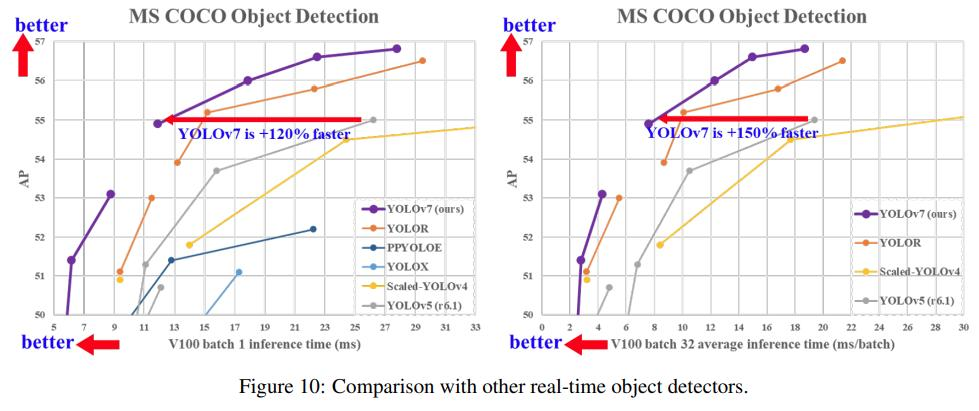YOLOV7整体网络结构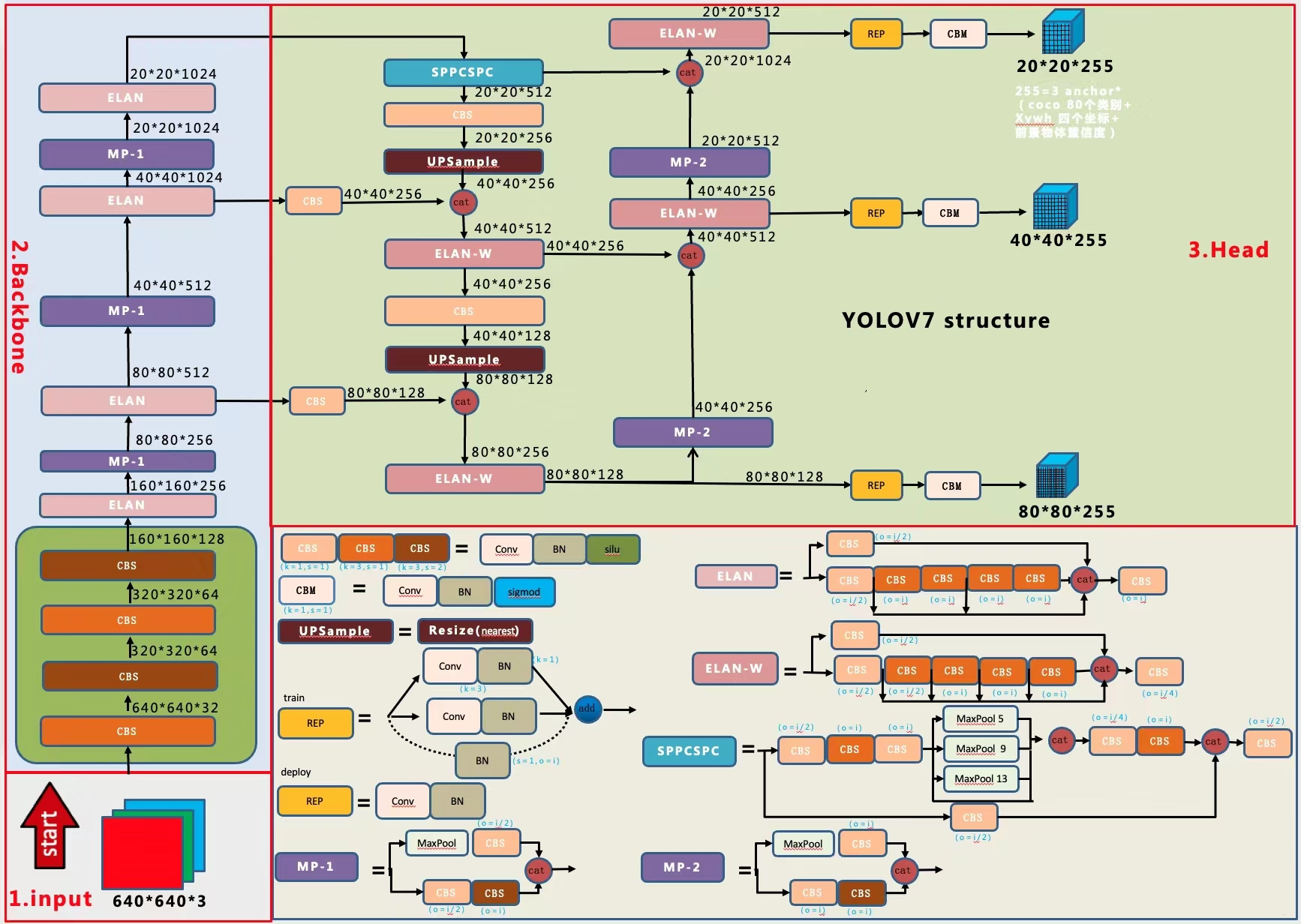class Conv(nn.Module):
# Standard convolution
def __init__(self, c1, c2, k=1, s=1, p=None, g=1, act=True):  # ch_in, ch_out, kernel, stride, padding, groups
super(Conv, self).__init__()
self.conv = nn.Conv2d(c1, c2, k, s, autopad(k, p), groups=g, bias=False)
self.bn = nn.BatchNorm2d(c2)
self.act = nn.SiLU() if act is True else (act if isinstance(act, nn.Module) else nn.Identity())

def forward(self, x):
return self.act(self.bn(self.conv(x)))

def fuseforward(self, x):
return self.act(self.conv(x))

# yolov7 backbone
backbone:
# [from, number, module, args]
[[-1, 1, Conv, [32, 3, 1]],  # 0

[-1, 1, Conv, [64, 3, 2]],  # 1-P1/2
[-1, 1, Conv, [64, 3, 1]],

[-1, 1, Conv, [128, 3, 2]],  # 3-P2/4  

YOLOV5在进一步的Backbone中使用的是C3结构，V7中改成了ELAN结构+MP结构。

• ELAN结构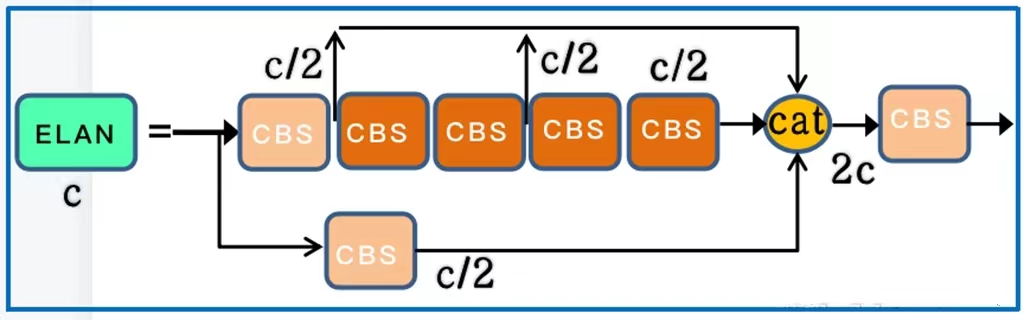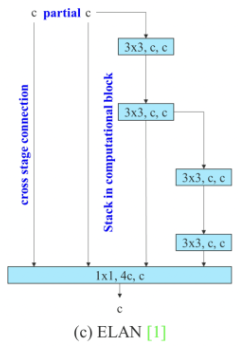class Concat(nn.Module):
def __init__(self, dimension=1):
super(Concat, self).__init__()
self.d = dimension

def forward(self, x):
return torch.cat(x, self.d)

[-1, 1, Conv, [64, 1, 1]],
[-2, 1, Conv, [64, 1, 1]],
[-1, 1, Conv, [64, 3, 1]],
[-1, 1, Conv, [64, 3, 1]],
[-1, 1, Conv, [64, 3, 1]],
[-1, 1, Conv, [64, 3, 1]],
[[-1, -3, -5, -6], 1, Concat, ],
[-1, 1, Conv, [256, 1, 1]],  # 11
• MP-1结构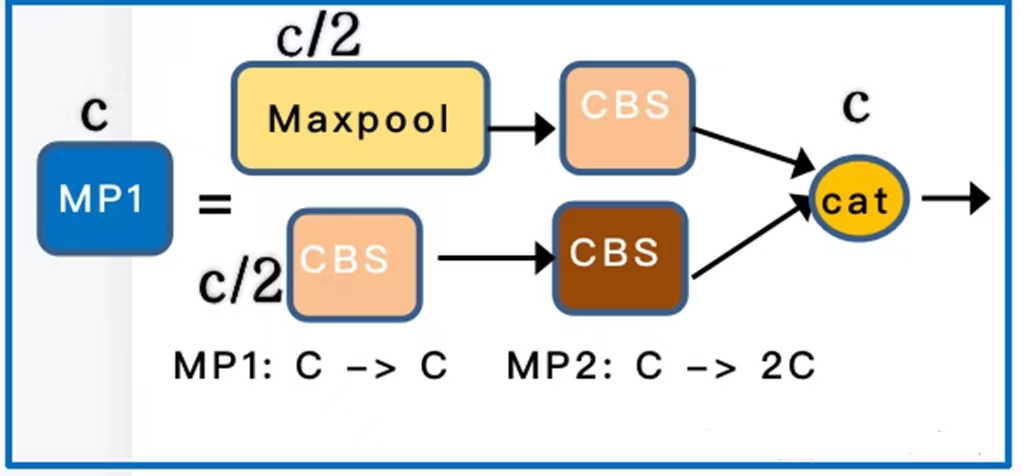class MP(nn.Module):
def __init__(self, k=2):
super(MP, self).__init__()
self.m = nn.MaxPool2d(kernel_size=k, stride=k)

def forward(self, x):
return self.m(x)

[-1, 1, MP, []],
[-1, 1, Conv, [128, 1, 1]],
[-3, 1, Conv, [128, 1, 1]],
[-1, 1, Conv, [128, 3, 2]],
[[-1, -3], 1, Concat, ],  # 16-P3/8  

YOLOV7的Backbone最深层的网络输出的特征图会经过一个叫SPPCSPC的结构再输入到Neck中，YOLOV5中则是SPPF的结构

• SPPCSPC结构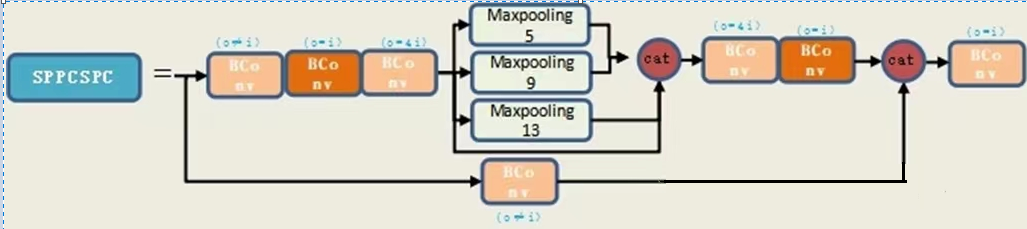class SPPCSPC(nn.Module):
# CSP https://github.com/WongKinYiu/CrossStagePartialNetworks
def __init__(self, c1, c2, n=1, shortcut=False, g=1, e=0.5, k=(5, 9, 13)):
super(SPPCSPC, self).__init__()
c_ = int(2 * c2 * e)  # hidden channels
self.cv1 = Conv(c1, c_, 1, 1)
self.cv2 = Conv(c1, c_, 1, 1)
self.cv3 = Conv(c_, c_, 3, 1)
self.cv4 = Conv(c_, c_, 1, 1)
self.m = nn.ModuleList([nn.MaxPool2d(kernel_size=x, stride=1, padding=x // 2) for x in k])
self.cv5 = Conv(4 * c_, c_, 1, 1)
self.cv6 = Conv(c_, c_, 3, 1)
self.cv7 = Conv(2 * c_, c2, 1, 1)

def forward(self, x):
x1 = self.cv4(self.cv3(self.cv1(x)))
y1 = self.cv6(self.cv5(torch.cat([x1] + [m(x1) for m in self.m], 1)))
y2 = self.cv2(x)
return self.cv7(torch.cat((y1, y2), dim=1))

# yolov7 head
[[-1, 1, SPPCSPC, ], # 51

Neck部分

YOLOV7依然是一个分层输出的特征金字塔结构。Backbone在第2、3、4个ELAN后进行特征分层输出。Neck部分依然采用的是PAN的双向特征融合，从深层到浅层进行上采样，再从浅层到深层进行下采样。PAN的上采样部分主要有CBS普通卷积，UpSample反卷积和ELAN-W的结构。

• ELAN-W结构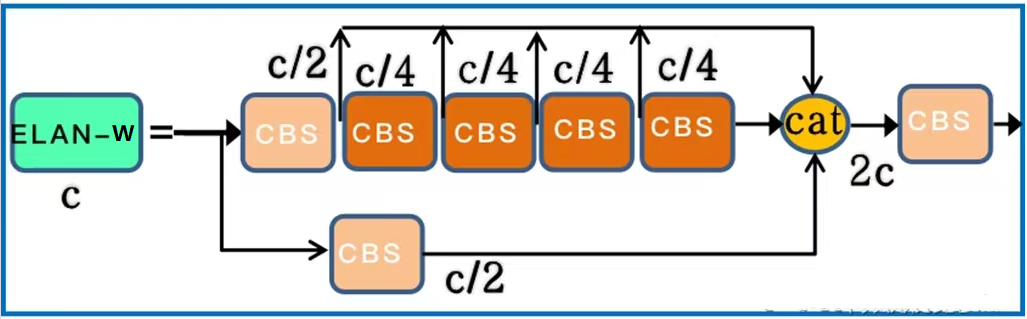ELAN-W跟ELAN基本上是一样的，但是它的concat合并是6层合并，ELAN是4层合并。配置文件如下

[-1, 1, Conv, [256, 1, 1]],
[-2, 1, Conv, [256, 1, 1]],
[-1, 1, Conv, [128, 3, 1]],
[-1, 1, Conv, [128, 3, 1]],
[-1, 1, Conv, [128, 3, 1]],
[-1, 1, Conv, [128, 3, 1]],
[[-1, -2, -3, -4, -5, -6], 1, Concat, ],
[-1, 1, Conv, [256, 1, 1]], # 63

PAN的下采样部分包括ELAN-W和MP-2两种结构

• MP-2结构MP-2结构和MP-1结构是一样的，它们的区别在于输出通道数不同，MP-2的输出通道数是MP-1的2倍。配置文件如下

[-1, 1, MP, []],
[-1, 1, Conv, [256, 1, 1]],
[-3, 1, Conv, [256, 1, 1]],
[-1, 1, Conv, [256, 3, 2]],
[[-1, -3, 51], 1, Concat, ],

• REP结构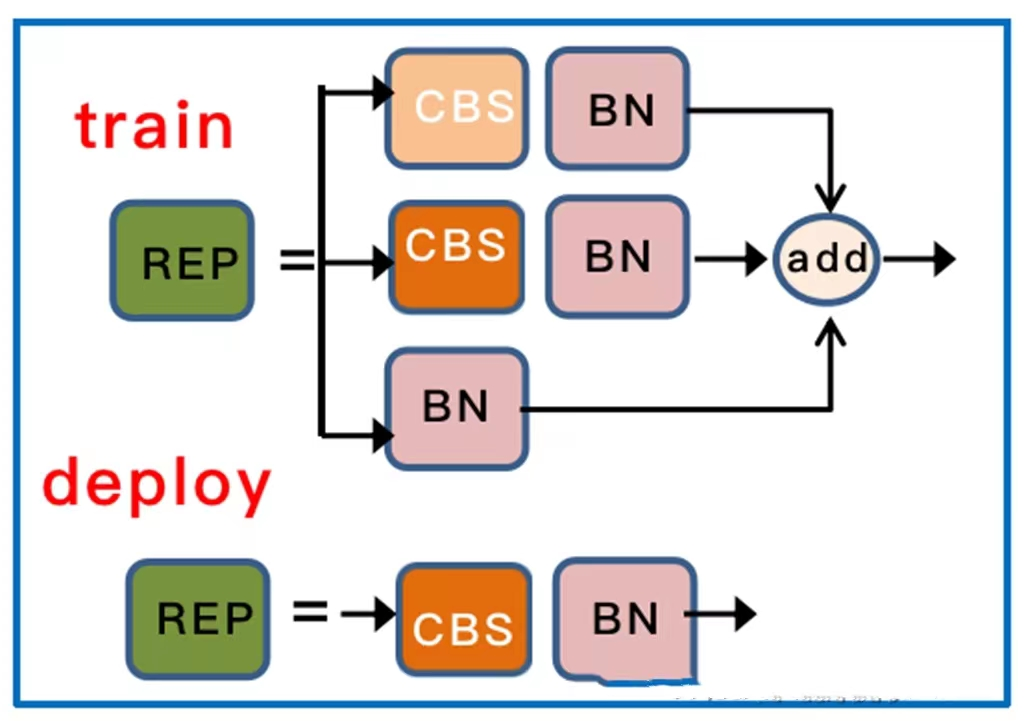REP在部署和训练的时候结构不同。在训练的时候，如果输入输出通道数相同，则包含了第三层BN的直连通道，否则只有第一层的1*1卷积+BN以及第二层的3*3卷积+BN，上面虽然画的是CBS，但其实这里是没有激活函数SiLu的，只有三层相加才会被最后激活。在部署时，为了方便部署，会将分支的参数重参数化到主分支上，取3*3的主分支卷积输出，但这里是没有BN的，只有激活。所以上面这个图是存在歧义的，需要指出。

class RepConv(nn.Module):
# Represented convolution
# https://arxiv.org/abs/2101.03697

def __init__(self, c1, c2, k=3, s=1, p=None, g=1, act=True, deploy=False):
super(RepConv, self).__init__()

self.deploy = deploy
self.groups = g
self.in_channels = c1
self.out_channels = c2

assert k == 3

self.act = nn.SiLU() if act is True else (act if isinstance(act, nn.Module) else nn.Identity())

if deploy:
# 如果是部署，则直接使用3*3的卷积
self.rbr_reparam = nn.Conv2d(c1, c2, k, s, autopad(k, p), groups=g, bias=True)

else:  # 如果是训练
# 如果输入输出通道数相同，则进行BatchNorm的直连
self.rbr_identity = (nn.BatchNorm2d(num_features=c1) if c2 == c1 and s == 1 else None)
# 3*3卷积+BatchNorm
self.rbr_dense = nn.Sequential(
nn.Conv2d(c1, c2, k, s, autopad(k, p), groups=g, bias=False),
nn.BatchNorm2d(num_features=c2),
)
# 1*1卷积+BatchNorm
self.rbr_1x1 = nn.Sequential(
nn.Conv2d( c1, c2, 1, s, padding_11, groups=g, bias=False),
nn.BatchNorm2d(num_features=c2),
)

def forward(self, inputs):
if hasattr(self, "rbr_reparam"):
return self.act(self.rbr_reparam(inputs))

if self.rbr_identity is None:
id_out = 0
else:
id_out = self.rbr_identity(inputs)

return self.act(self.rbr_dense(inputs) + self.rbr_1x1(inputs) + id_out)

[75, 1, RepConv, [256, 3, 1]],
[88, 1, RepConv, [512, 3, 1]],
[101, 1, RepConv, [1024, 3, 1]],
• 模型的重参化

RepConv我们需要看成一个整体，重参化的意思就是它相比于一个普通的卷积，因为有三层结构，它的参数量更多了，也更适合于反向传播。它在VGG上取得了优异的性能，但将它直接用于ResNet和DenseNet时，它的精度反而会显著下降。作者使用梯度传播路径来分析不同的重参化模块应该和哪些网络搭配使用。通过分析RepConv与不同架构的组合以及产生的性能，发现RepConv中的identity破坏了ResNet中的残差结构和DenseNet中的跨层连接，这为不同的特征图提供了梯度的多样性。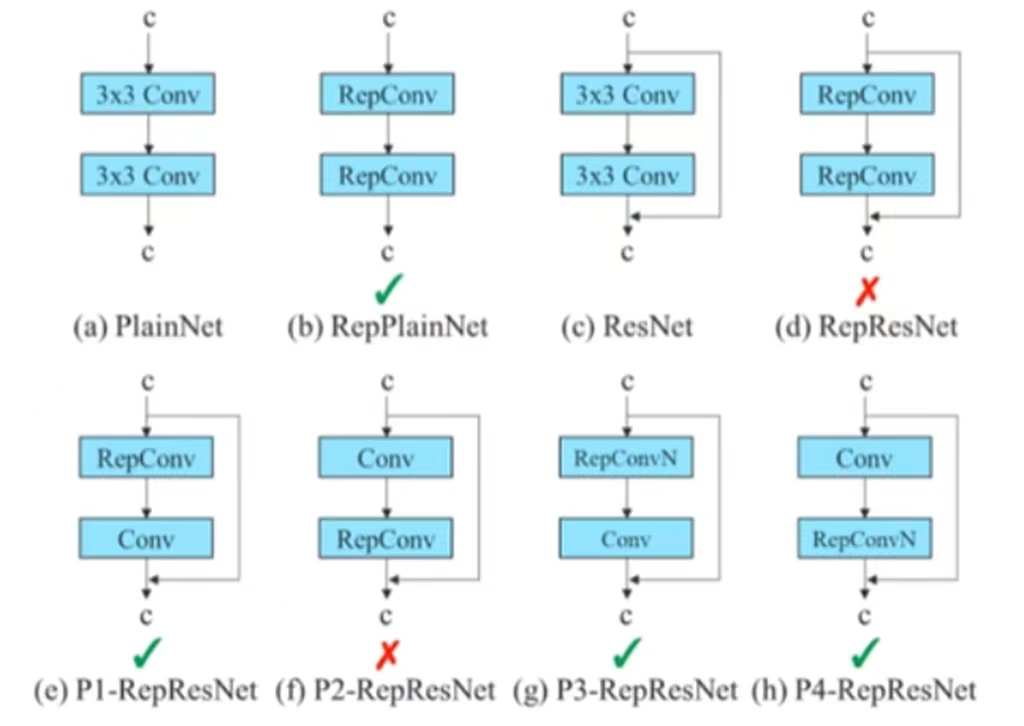• 辅助训练模块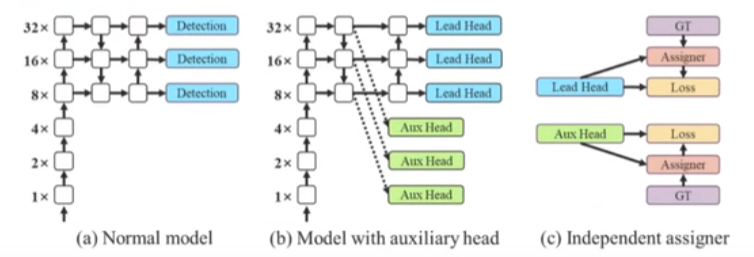Coarse-to-fine lead guided label assigner(粗到细引导标签分配器):Coarse-to-fine引导头使用到了自身的prediction和ground truth来生成软标签，引导标签进行分配。然而在这个过程中，作者生成了两组不同的软标签，即粗标签和细标签。其中细标签与引导头在标签分配器上生成的软标签相同，粗标签是通过降低正样本分配的约束，允许更多的网络作为正目标。原因是一个辅助头的学习能力并不需要强大的引导头，为了避免丢失信息，作者将专注于优化样本召回的辅助头。对于引导头的输出，可以从查准率中过滤出高精度值的结果作为最终输出。然而，值得注意的是，如果粗标签的附加权值接近细标签的附加权值，则可能会在最终预测时产生错误的先验结果。### 作者的其它热门文章

0
0 收藏

0 评论
0 收藏
0